# Search ResultsCollection Resources(1009)

View
Selected filters:
• GeometryConditions of Use:
Remix and Share
Rating

This task examines the ways in which the plane can be covered ...

This task examines the ways in which the plane can be covered by regular polygons in a very strict arrangement called a regular tessellation. These tessellations are studied here using algebra, which enters the picture via the formula for the measure of the interior angles of a regular polygon (which should therefore be introduced or reviewed before beginning the task). The goal of the task is to use algebra in order to understand which tessellations of the plane with regular polygons are possible.

Subject:
Mathematics
Algebra
Geometry
Material Type:
Activity/Lab
Provider:
Illustrative Mathematics
Provider Set:
Illustrative Mathematics
Author:
Illustrative Mathematics
Date created
3-جمادي الأولى-1434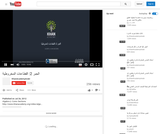Conditions of Use:
Remix and Share
Rating

This 14-minute video lesson looks at conic sections.

Subject:
Algebra
Geometry
Material Type:
Lecture
Provider:
Provider Set:
Author:
Khan, Salman
Date created
25-ذو القعدة-1433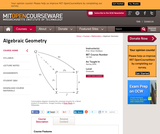Conditions of Use:
Remix and Share
Rating

" This course provides an introduction to the language of schemes, properties ...

" This course provides an introduction to the language of schemes, properties of morphisms, and sheaf cohomology. Together with 18.725 Algebraic Geometry, students gain an understanding of the basic notions and techniques of modern algebraic geometry."

Subject:
Algebra
Geometry
Material Type:
Full Course
Textbook
Provider:
M.I.T.
Provider Set:
M.I.T. OpenCourseWare
Author:
Kedlaya, Kiran
Date created
13-ربيع الأول-1432Conditions of Use:
Remix and Share
Rating

Equations of a Straight Line

Subject:
Geometry
Material Type:
Lecture
Provider:
University of Tabuk
Author:
University of Tabuk
محمد عادل صنوفي
Date created
7-جمادي الآخرة-1440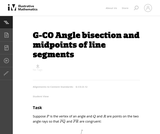Conditions of Use:
Remix and Share
Rating

This task provides a construction of the angle bisector of an angle ...

This task provides a construction of the angle bisector of an angle by reducing it to the bisection of an angle to finding the midpoint of a line segment. It is worth observing the symmetry -- for both finding midpoints and bisecting angles, the goal is to cut an object into two equal parts. The conclusion of this task is that they are, in a sense, of exactly equivalent difficulty -- bisecting a segment allows us to bisect and angle (part a) and, conversely, bisecting an angle allows us to bisect a segment (part b). In addition to seeing how these two constructions are related, the task also provides an opportunity for students to use two different triangle congruence criteria: SSS and SAS.

Subject:
Mathematics
Geometry
Material Type:
Activity/Lab
Provider:
Illustrative Mathematics
Provider Set:
Illustrative Mathematics
Author:
Illustrative Mathematics
Date created
3-جمادي الأولى-1434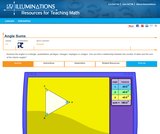Conditions of Use:
Rating

With this applet, students can examine the angles in a triangle, quadrilateral, ...

With this applet, students can examine the angles in a triangle, quadrilateral, pentagon, hexagon, heptagon or octagon. They can change the shape of the figure by dragging the vertices; the size of each angle is shown and the sum of the interior angles calculated. Students are challenged to find a relationship between the number of sides and the sum of the interior angles.

Subject:
Geometry
Material Type:
Interactive
Lesson Plan
Provider:
National Council of Teachers of Mathematics
Provider Set:
Illuminations
Date created
20-شوال-1433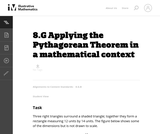Conditions of Use:
Remix and Share
Rating

This task requires students to apply the Pythagorean Theorem.

Subject:
Mathematics
Geometry
Material Type:
Activity/Lab
Provider:
Illustrative Mathematics
Provider Set:
Illustrative Mathematics
Author:
Illustrative Mathematics
Date created
1-جمادي الأولى-1434Rating

In this math activity, learners explore the history of the Stomachion (an ...

In this math activity, learners explore the history of the Stomachion (an ancient tangram-type puzzle), use the pieces to create other figures, learn about symmetry and transformations, and investigate the areas of the pieces. The Stomachion, believed to have been created by Archimedes, consists of 14 pieces cut from a square, which can be rearranged to form other interesting shapes.

Subject:
Mathematics
Geometry
Material Type:
Interactive
Lesson Plan
Provider:
National Council of Teachers of Mathematics
Provider Set:
Illuminations
Author:
NCTM Illuminations
Samuel E. Zordak
Thinkfinity/Verizon Foundation
Date created
17-محرم-1436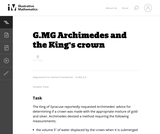Conditions of Use:
Remix and Share
Rating

The famous story of Archimedes running through the streets of Syracuse (in ...

The famous story of Archimedes running through the streets of Syracuse (in Sicily during the third century bc) shouting ''Eureka!!!'' (I have found it) reportedly occurred after he solved this problem. The problem combines the ideas of ratio and proportion within the context of density of matter.

Subject:
Mathematics
Geometry
Material Type:
Activity/Lab
Provider:
Illustrative Mathematics
Provider Set:
Illustrative Mathematics
Author:
Illustrative Mathematics
Date created
3-جمادي الأولى-1434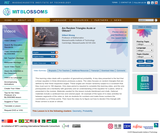Conditions of Use:
Remix and Share
Rating

This learning video deals with a question of geometrical probability. A key ...

This learning video deals with a question of geometrical probability. A key idea presented is the fact that a linear equation in three dimensions produces a plane. The video focuses on random triangles that are defined by their three respective angles. These angles are chosen randomly subject to a constraint that they must sum to 180 degrees. An example of the types of in-class activities for between segments of the video is: Ask six students for numbers and make those numbers the coordinates x,y of three points. Then have the class try to figure out how to decide if the triangle with those corners is acute or obtuse.

Subject:
Geometry
Statistics and Probability
Material Type:
Lecture
Provider:
M.I.T.
Provider Set:
M.I.T. Blossoms
Author:
Gilbert Strange
Date created
12-رجب-1433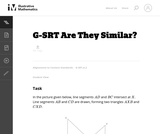Conditions of Use:
Remix and Share
Rating

In this problem, students are given a picture of two triangles that ...

In this problem, students are given a picture of two triangles that appear to be similar, but whose similarity cannot be proven without further information. Asking students to provide a sequence of similarity transformations that maps one triangle to the other focuses them on the work of standard G-SRT.2, using the definition of similarity in terms of similarity transformations.

Subject:
Mathematics
Geometry
Trigonometry
Material Type:
Activity/Lab
Provider:
Illustrative Mathematics
Provider Set:
Illustrative Mathematics
Author:
Illustrative Mathematics
Date created
3-جمادي الأولى-1434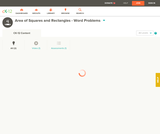Conditions of Use:
Remix and Share
Rating

This short video and interactive assessment activity is designed to teach fourth ...

This short video and interactive assessment activity is designed to teach fourth graders about area of squares and rectangles - word problems.

Subject:
Mathematics
Geometry
Material Type:
Assessment
Interactive
Lecture
Provider:
CK-12 Foundation
Provider Set:
CK-12 Elementary Math
Date created
15-جمادي الأولى-1436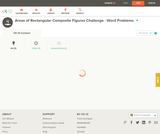Conditions of Use:
Remix and Share
Rating

This short video and interactive assessment activity is designed to teach fourth ...

This short video and interactive assessment activity is designed to teach fourth graders about areas of rectangular composite figures challenge - word problems.

Subject:
Mathematics
Geometry
Material Type:
Assessment
Interactive
Lecture
Provider:
CK-12 Foundation
Provider Set:
CK-12 Elementary Math
Date created
15-جمادي الأولى-1436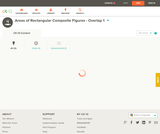Conditions of Use:
Remix and Share
Rating

This short video and interactive assessment activity is designed to teach fourth ...

This short video and interactive assessment activity is designed to teach fourth graders about areas of rectangular composite figures - overlap 1.

Subject:
Mathematics
Geometry
Material Type:
Assessment
Interactive
Lecture
Provider:
CK-12 Foundation
Provider Set:
CK-12 Elementary Math
Date created
15-جمادي الأولى-1436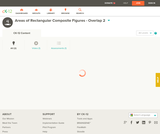Conditions of Use:
Remix and Share
Rating

This short video and interactive assessment activity is designed to teach fourth ...

This short video and interactive assessment activity is designed to teach fourth graders about areas of rectangular composite figures - overlap 2.

Subject:
Mathematics
Geometry
Material Type:
Assessment
Interactive
Lecture
Provider:
CK-12 Foundation
Provider Set:
CK-12 Elementary Math
Date created
15-جمادي الأولى-1436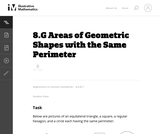Conditions of Use:
Remix and Share
Rating

This problem is part of a very rich tradition of problems looking ...

This problem is part of a very rich tradition of problems looking to maximize the area enclosed by a shape with fixed perimeter. Only three shapes are considered here because the problem is difficult for more irregular shapes.

Subject:
Mathematics
Geometry
Material Type:
Activity/Lab
Provider:
Illustrative Mathematics
Provider Set:
Illustrative Mathematics
Author:
Illustrative Mathematics
Date created
1-جمادي الأولى-1434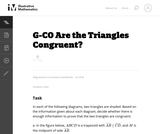Conditions of Use:
Remix and Share
Rating

The purpose of this task is primarily assessment-oriented, asking students to demonstrate ...

The purpose of this task is primarily assessment-oriented, asking students to demonstrate knowledge of how to determine the congruency of triangles.

Subject:
Mathematics
Geometry
Material Type:
Activity/Lab
Provider:
Illustrative Mathematics
Provider Set:
Illustrative Mathematics
Author:
Illustrative Mathematics
Date created
3-جمادي الأولى-1434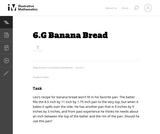Conditions of Use:
Remix and Share
Rating

The purpose of this task is to provide students with a multi-step ...

The purpose of this task is to provide students with a multi-step problem involving volume and to give them a chance to discuss the difference between exact calculations and their meaning in a context.

Subject:
Mathematics
Geometry
Material Type:
Activity/Lab
Provider:
Illustrative Mathematics
Provider Set:
Illustrative Mathematics
Author:
Illustrative Mathematics
Date created
29-ربيع الثاني-1434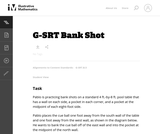Conditions of Use:
Remix and Share
Rating

This task asks students to use similarity to solve a problem in ...

This task asks students to use similarity to solve a problem in a context that will be familiar to many, though most students are accustomed to using intuition rather than geometric reasoning to set up the shot.

Subject:
Mathematics
Geometry
Trigonometry
Material Type:
Activity/Lab
Provider:
Illustrative Mathematics
Provider Set:
Illustrative Mathematics
Author:
Illustrative Mathematics
Date created
3-جمادي الأولى-1434